|

# 有整容的钱,还不如买这些!

在品味面前，颜值也要屈居次席，好多看着“光鲜亮丽”的型男，手边的包里各种东西纠缠在一起，实在是让人大跌眼镜。往往我们随身携带的物件细节就是彰显品味的关键。理想的情况无非是每个从包包里掏出的东西，比如皮夹、钥匙圈、名片盒、手帕等等这些随身物件，都能以低调自然的样貌给旁人留下深刻印象。相信有了下面这些产品的加持，也能让好品味自然而然流露于你的举手投足间——

包包总是乱糟糟？This is ground让你懂得收纳的品味

身边的数码产品越来越多，但随之而来的就是它们的收纳问题，各种数据线纠缠在一起，到需要用的时候，真的是好不痛苦。

来自美国的品牌 This is ground，就为此带来了各式不同的集线器、皮革收纳包，并且坚持所有产品都在洛杉矶制造。每款包里更是有多个小小的隔间可放不同的线材，这样贴心的设计，让时常要带着科技产品出门的人们有个更有条理的收纳方式，所有所需工具都在打开包包后一目了然。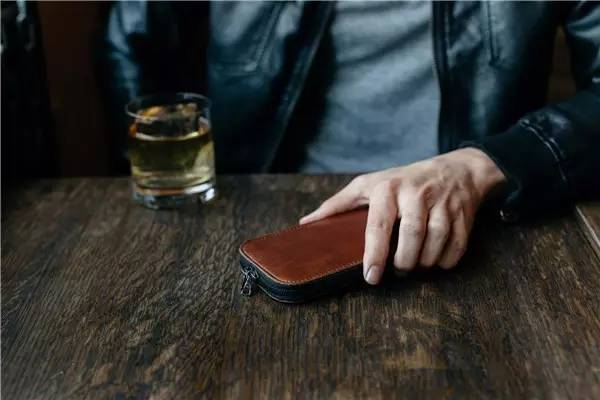变换自如的智能行李箱 Bugaboo Boxer

在旅行中，一个方便好用的行李箱将会让旅途更舒适。在许多时候我们会发现推着行李箱才是最合适的移动形式，尤其赶路时后头的行李箱总是阻碍行动。而这款Bugaboo Boxer就可以自由选择推行或是拖拉的方式移动。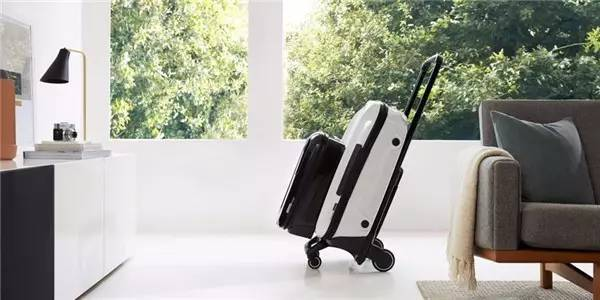可以先通过下面这个视频去感受这款行李箱的实用和酷炫。

它主要由附有车轮的轻钢架组成，可以根据旅行的需求变化不同模式加装或减少收纳箱，随身贵重物品可以放在最内层也不用担心取用时不方便，分离式的系统收纳将一切都变得简单，可以说是一款「智能型行李箱」。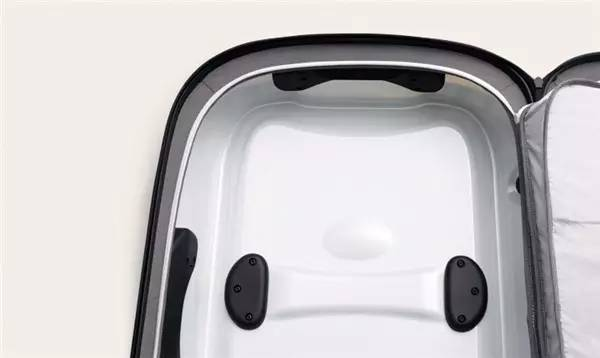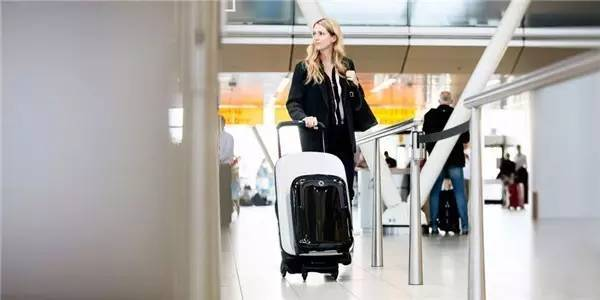经典重塑，德国博朗将在今年重现两款经典腕表作

BRAUN 的经典不用多说，这次要来谈的是 Dieter Rams 在较晚期为 BRAUN 设计的手表，因为就在今年，BRAUN 两大经典手表作 AW10 与 AW50 即将复刻登场。BRAUN 在 1977 年才诞生手表这条产品线，由 Dieter Rams 和 Dietrich Lubs 共同操刀，起初两位对于手表设计并非立即上手，尽管 BRAUN 做过各式各样家电产品，但要在手表这个严格来说不算电器的「饰品」上，注入 BRAUN 重视的简洁和干净线条感，实属一大考验。这是他们最早推出的作品：DW20 & DW30，是电子表的规格：随后他们认为这虽能彰显 BRAUN 是做电器出身的品牌，却少了他们想追求的经典感。于是两人一致认同这不够 BRAUN，BRAUN 的精神是找出物件所能呈现的最纯粹与原始之态，留下该留的、舍去无用的。由此，便迎来经典表款的问世——AW10 & AW50：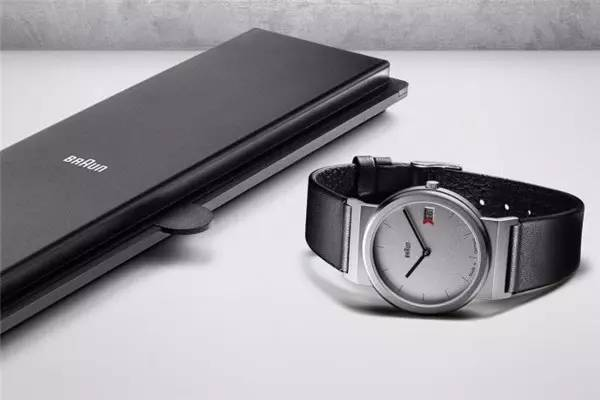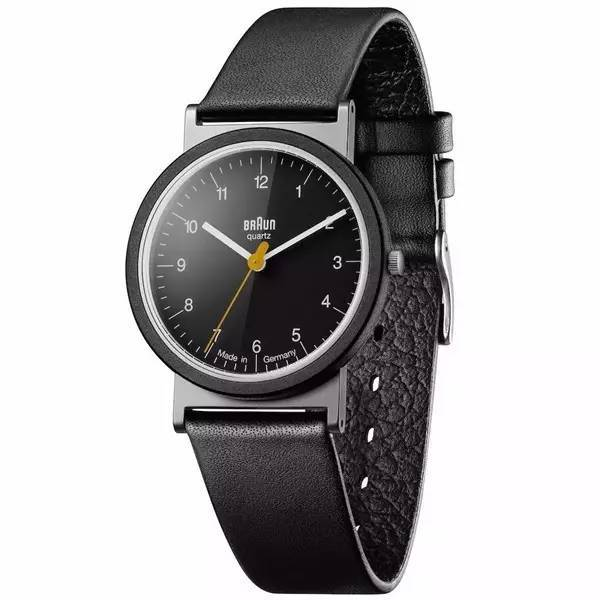尽管不像瑞士表那样强调精致机芯，也不像当时潮流所趋的电子表，但这样简约而单纯的「基本」样貌反让 BRAUN 在手表市场上占据独特的一角，也让我们期待起它们的回归。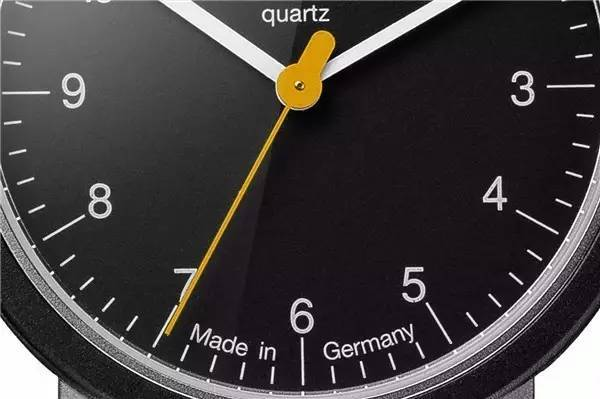一点细节都不能放过，卫生纸也成为时尚配件

我们注重皮夹、钥匙圈、名片盒等等随身小物的美感，不过有个东西我们绝对不会去在意 — 那就是卫生纸。如果你也曾认为包包里的面纸拿出来跟自己很不搭，如果你也曾因找不到好看的面纸而困扰，那么 Renova 这个居家纸品品牌或许能为你解决问题。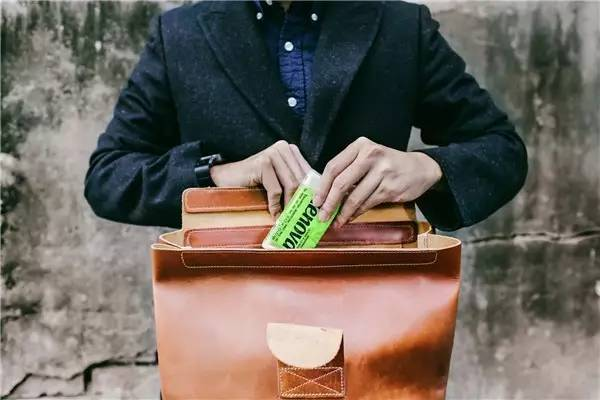来自葡萄牙的 Renova，品牌名称为拉丁文「创新」之意，从 1939 年成立至今已有 80 年历史，2005 年 Renova 以颠覆传统的思维推出黑色纸巾，新颖的个性引起热烈回响，随后他们更接连推出各种的缤纷色彩，让原先再平凡不过的日用品换上不同样貌。随后更是席卷时尚界与各大媒体，跃升欧洲消费者心中高级用纸的第一首选，甚至有「卫生纸界的爱马仕」之称。设计好看的包装、多元多样的选色，拿出面纸的瞬间也是充满自信、展现风格的时刻有没有。也许有人看到彩色纸巾会担心健康问题，不过 Renova 在这点上非常强调它们用的是纯植物染料，没有荧光剂也不添加重金属或漂白剂等有害物质，是 100% 纯天然原生纸浆打造的舒服厚实质地，对讲究面纸触感的人而言非常适合。这款「极简化」信用卡，就算没有刷卡习惯的你也想要收藏

近期，英国银行 Revolut 和设计公司 Blond 联名合作，共同推出一款「极简化」信用卡，没有显眼的银行 Logo、个人资料以及账号数字，留下的是，右上角的银行标志「R」以及左下角的用户姓名，如此干净又素面的色调质感、极简化的风格，和市面上五颜六色的卡片区隔开来。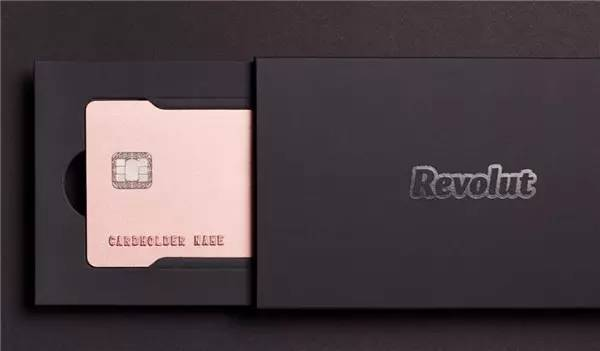除了大幅提升了外观的封面质感，该公司还特别注意到客户最在意的安全隐私，并将账号数字、有效日期…等信息，纷纷隐藏至卡片背后，让你在刷卡使用的同时，不用再担心身旁人注视的眼光。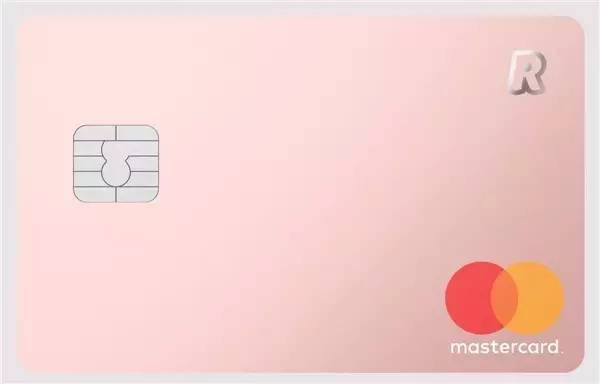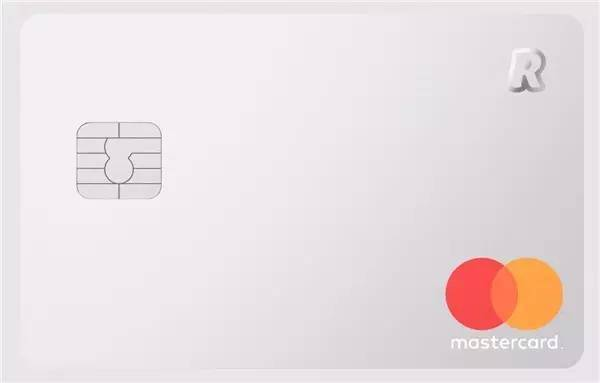像机车一样帅的锂电自行车「Super 73」

创立于加州的 lithium cycles 团队，希望推出一款像机车般经典帅气、又具备现代化科技的电动自行车，让注重环保议题的繁忙都市人，在交通上拥有更便捷的选择，于是突破以往 e-bike 设计的「Super 73 」就这么诞生了。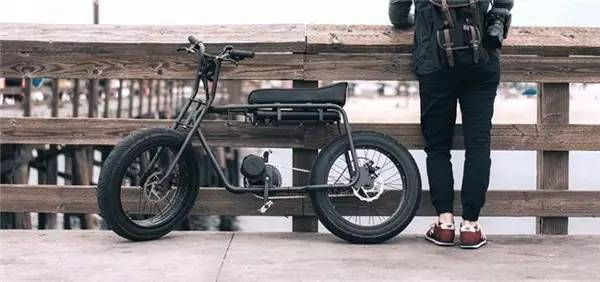Super 73 的外观留有机车的元素，结构部分则融入现代极简风的前卫感，搭上锂电池装置，续航可达 32 公里之久，时速则可以达到每小时 48 公里。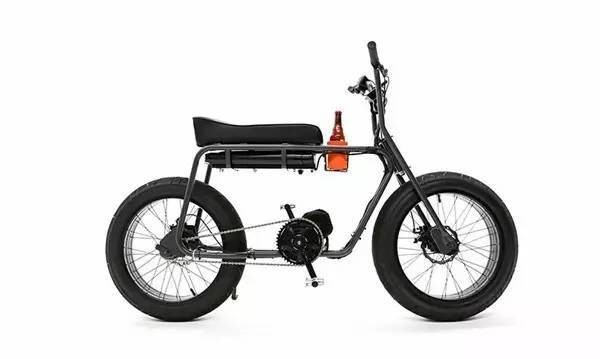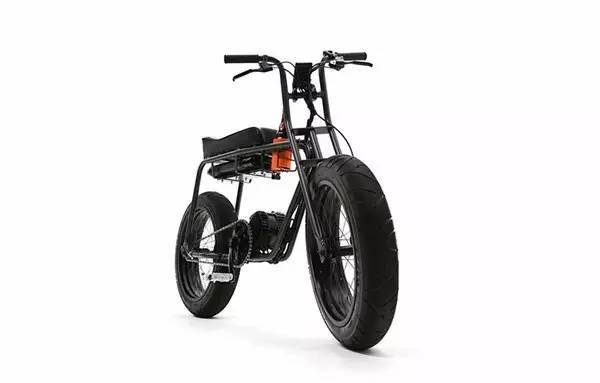为了安全考量还做了急煞盘式制动器系统，让车子在煞车时会自动关闭引擎、安稳停下，握把处也设有马达控制键，让骑乘者随时调节车速。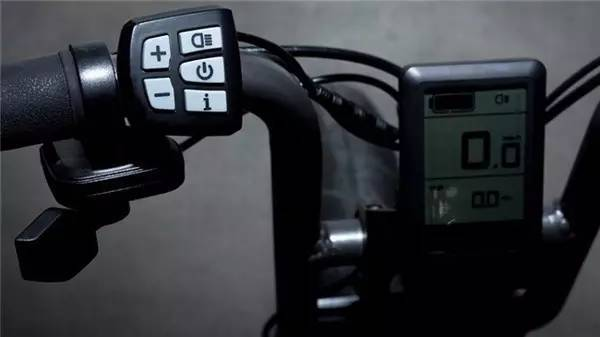Super 73 是透过 USB 充电，这项装置也让手机能在骑车的同时充电，相当贴心。坐垫前方有饮料杯架。而杯架前面还有个开瓶器装置，可以开一些…不是酒的瓶子。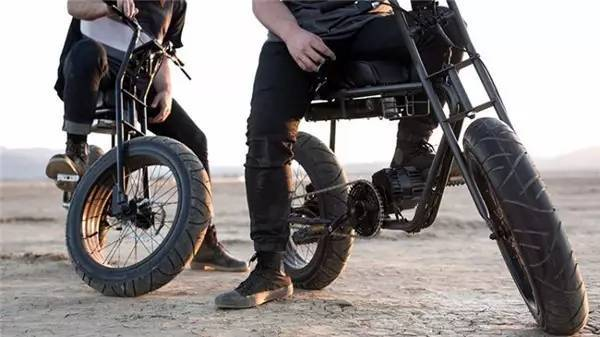细节成就你的风格 Candy Design & Works

无论是身上的衬衫缝线、剪裁，裙子的长度、版型，或甚至使用的皮带、钥匙圈等等，这些零碎的细节与物件，往往是成就风格、展现品味最重要的元素。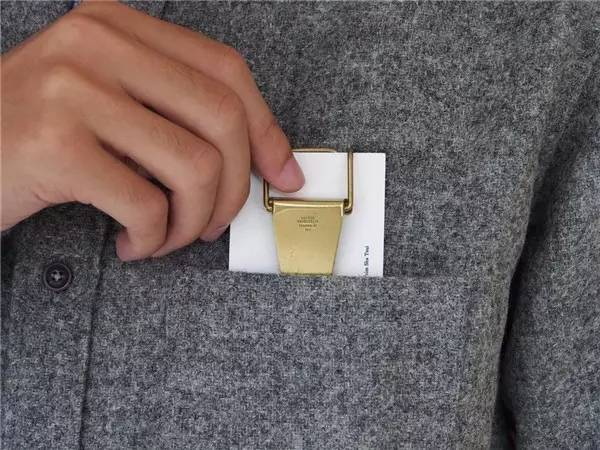由日本设计师川本治朗（Jiro Kawamoto）于 2010年成立的Candy Design & Works ，源自于川本先生对上世纪 20 至 30 年代的古董小物情有独钟，运用当时的设计及传统工艺制作各种饰物。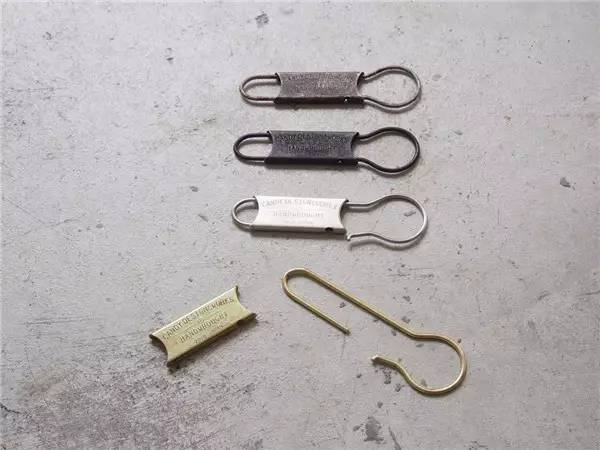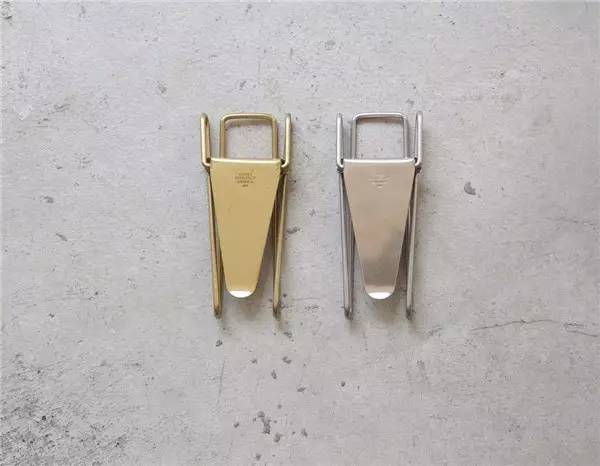无论是是考究 1890 年代的原形，以圆弧形的造型与圆形转动盘为主轴的设计；亦或是配合男士或中性风格使的扣环设计可系在裤头的皮带环；以及采用日本的古老月份，睦月、如月、弥生…等12个月做划分，充满纪念价值的月份钥匙圈。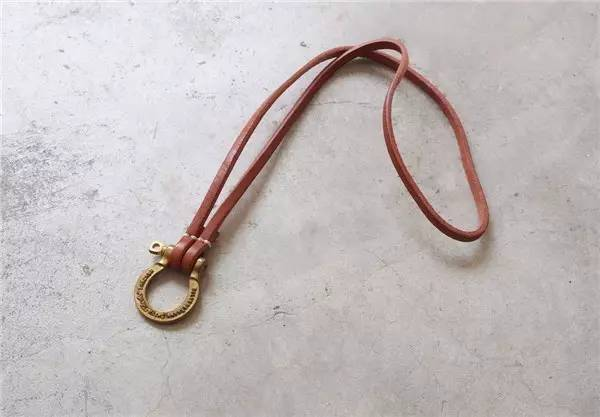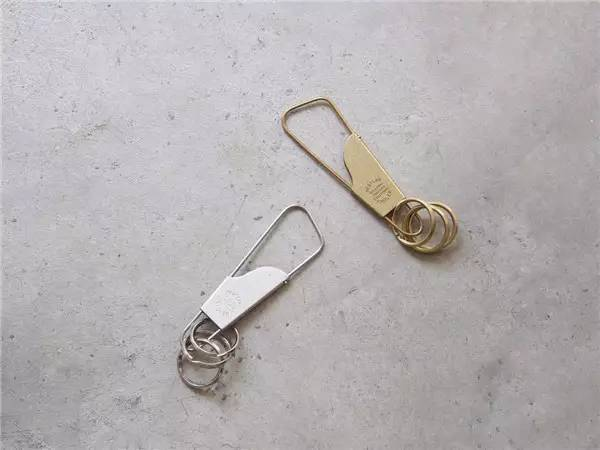金属铜制材质，复古精确设计，无论是握在手中或系在腰间都独具个人风味。让制笔界的艺术家Georg Jensen Men’s 为你的气质加分

「笔」这项工具已经不单只是书写用途，更是一个品味的象征，Georg Jensen Men’s 钻研于简约的设计，并在男性配件上做出绅士与现代共存的完美设计。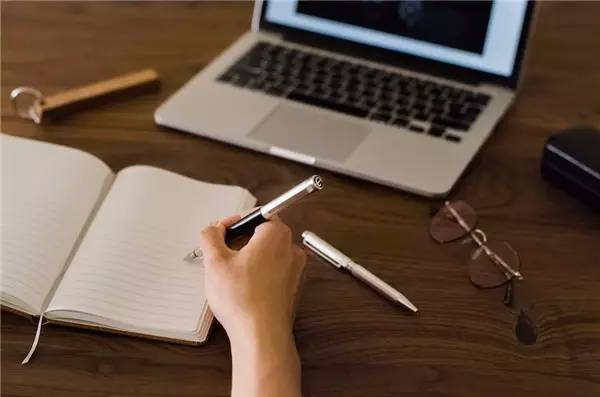Georg Jensen Men’s 将工艺技法注入于笔款设计上，看似简约的线条框架下却藏有迷人的色泽，运用纯银精制的笔身经过细琢后，在挥笔的每一个角度都散发着沉稳的气息。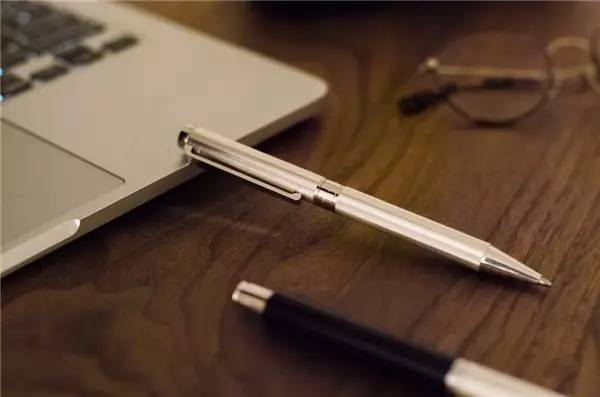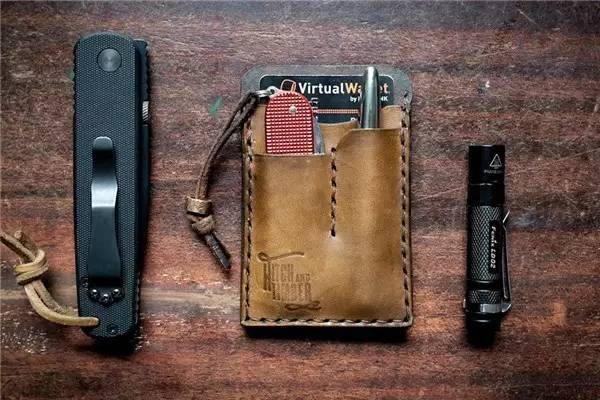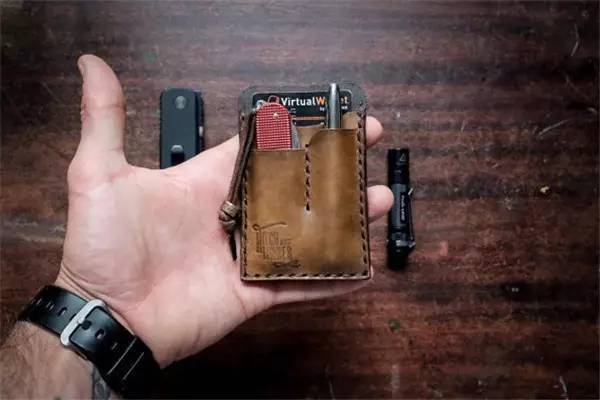EDC即「Everyday carry」的缩写，简单而言就是每日必带的东西，融合钱包与卡夹的功能，最多能装下五张卡片，并能同时容纳如钢笔、原子笔、小刀等随身小物，且外型大小适中，可以刚好放进牛仔裤或衬衫口袋。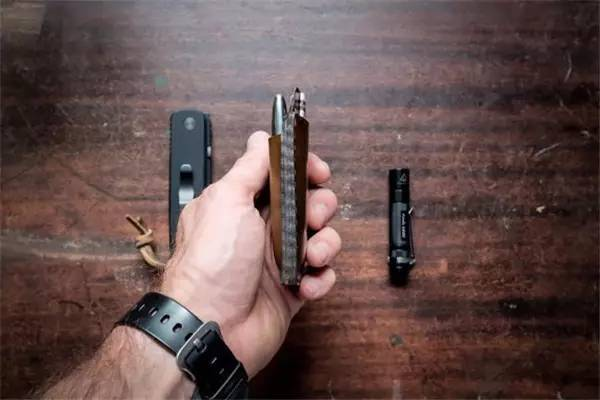`声明：本文由入驻焦点开放平台的作者撰写，除焦点官方账号外，观点仅代表作者本人，不代表焦点立场错误信息举报电话： 400-099-0099，邮箱：jubao@vip.sohu.com，或点此进行意见反馈，或点此进行举报投诉。`A B C D E F G H J K L M N P Q R S T W X Y Z
A - B - C - D - E
• A
• 鞍山
• 安庆
• 安阳
• 安顺
• 安康
• 澳门
• B
• 北京
• 保定
• 包头
• 巴彦淖尔
• 本溪
• 蚌埠
• 亳州
• 滨州
• 北海
• 百色
• 巴中
• 毕节
• 保山
• 宝鸡
• 白银
• 巴州
• C
• 承德
• 沧州
• 长治
• 赤峰
• 朝阳
• 长春
• 常州
• 滁州
• 池州
• 长沙
• 常德
• 郴州
• 潮州
• 崇左
• 重庆
• 成都
• 楚雄
• 昌都
• 慈溪
• 常熟
• D
• 大同
• 大连
• 丹东
• 大庆
• 东营
• 德州
• 东莞
• 德阳
• 达州
• 大理
• 德宏
• 定西
• 儋州
• 东平
• E
• 鄂尔多斯
• 鄂州
• 恩施
F - G - H - I - J
• F
• 抚顺
• 阜新
• 阜阳
• 福州
• 抚州
• 佛山
• 防城港
• G
• 赣州
• 广州
• 桂林
• 贵港
• 广元
• 广安
• 贵阳
• 固原
• H
• 邯郸
• 衡水
• 呼和浩特
• 呼伦贝尔
• 葫芦岛
• 哈尔滨
• 黑河
• 淮安
• 杭州
• 湖州
• 合肥
• 淮南
• 淮北
• 黄山
• 菏泽
• 鹤壁
• 黄石
• 黄冈
• 衡阳
• 怀化
• 惠州
• 河源
• 贺州
• 河池
• 海口
• 红河
• 汉中
• 海东
• 怀来
• I
• J
• 晋中
• 锦州
• 吉林
• 鸡西
• 佳木斯
• 嘉兴
• 金华
• 景德镇
• 九江
• 吉安
• 济南
• 济宁
• 焦作
• 荆门
• 荆州
• 江门
• 揭阳
• 金昌
• 酒泉
• 嘉峪关
K - L - M - N - P
• K
• 开封
• 昆明
• 昆山
• L
• 廊坊
• 临汾
• 辽阳
• 连云港
• 丽水
• 六安
• 龙岩
• 莱芜
• 临沂
• 聊城
• 洛阳
• 漯河
• 娄底
• 柳州
• 来宾
• 泸州
• 乐山
• 六盘水
• 丽江
• 临沧
• 拉萨
• 林芝
• 兰州
• 陇南
• M
• 牡丹江
• 马鞍山
• 茂名
• 梅州
• 绵阳
• 眉山
• N
• 南京
• 南通
• 宁波
• 南平
• 宁德
• 南昌
• 南阳
• 南宁
• 内江
• 南充
• P
• 盘锦
• 莆田
• 平顶山
• 濮阳
• 攀枝花
• 普洱
• 平凉
Q - R - S - T - W
• Q
• 秦皇岛
• 齐齐哈尔
• 衢州
• 泉州
• 青岛
• 清远
• 钦州
• 黔南
• 曲靖
• 庆阳
• R
• 日照
• 日喀则
• S
• 石家庄
• 沈阳
• 双鸭山
• 绥化
• 上海
• 苏州
• 宿迁
• 绍兴
• 宿州
• 三明
• 上饶
• 三门峡
• 商丘
• 十堰
• 随州
• 邵阳
• 韶关
• 深圳
• 汕头
• 汕尾
• 三亚
• 三沙
• 遂宁
• 山南
• 商洛
• 石嘴山
• T
• 天津
• 唐山
• 太原
• 通辽
• 铁岭
• 泰州
• 台州
• 铜陵
• 泰安
• 铜仁
• 铜川
• 天水
• 天门
• W
• 乌海
• 乌兰察布
• 无锡
• 温州
• 芜湖
• 潍坊
• 威海
• 武汉
• 梧州
• 渭南
• 武威
• 吴忠
• 乌鲁木齐
X - Y - Z
• X
• 邢台
• 徐州
• 宣城
• 厦门
• 新乡
• 许昌
• 信阳
• 襄阳
• 孝感
• 咸宁
• 湘潭
• 湘西
• 西双版纳
• 西安
• 咸阳
• 西宁
• 仙桃
• 西昌
• Y
• 运城
• 营口
• 盐城
• 扬州
• 鹰潭
• 宜春
• 烟台
• 宜昌
• 岳阳
• 益阳
• 永州
• 阳江
• 云浮
• 玉林
• 宜宾
• 雅安
• 玉溪
• 延安
• 榆林
• 银川
• Z
• 张家口
• 镇江
• 舟山
• 漳州
• 淄博
• 枣庄
• 郑州
• 周口
• 驻马店
• 株洲
• 张家界
• 珠海
• 湛江
• 肇庆
• 中山
• 自贡
• 资阳
• 遵义
• 昭通
• 张掖
• 中卫

1室1厅1厨1卫1阳台

1
2
3
4
5

0
1
2

1

1

0
1
2
3报名成功，资料已提交审核A B C D E F G H J K L M N P Q R S T W X Y Z
A - B - C - D - E
• A
• 鞍山
• 安庆
• 安阳
• 安顺
• 安康
• 澳门
• B
• 北京
• 保定
• 包头
• 巴彦淖尔
• 本溪
• 蚌埠
• 亳州
• 滨州
• 北海
• 百色
• 巴中
• 毕节
• 保山
• 宝鸡
• 白银
• 巴州
• C
• 承德
• 沧州
• 长治
• 赤峰
• 朝阳
• 长春
• 常州
• 滁州
• 池州
• 长沙
• 常德
• 郴州
• 潮州
• 崇左
• 重庆
• 成都
• 楚雄
• 昌都
• 慈溪
• 常熟
• D
• 大同
• 大连
• 丹东
• 大庆
• 东营
• 德州
• 东莞
• 德阳
• 达州
• 大理
• 德宏
• 定西
• 儋州
• 东平
• E
• 鄂尔多斯
• 鄂州
• 恩施
F - G - H - I - J
• F
• 抚顺
• 阜新
• 阜阳
• 福州
• 抚州
• 佛山
• 防城港
• G
• 赣州
• 广州
• 桂林
• 贵港
• 广元
• 广安
• 贵阳
• 固原
• H
• 邯郸
• 衡水
• 呼和浩特
• 呼伦贝尔
• 葫芦岛
• 哈尔滨
• 黑河
• 淮安
• 杭州
• 湖州
• 合肥
• 淮南
• 淮北
• 黄山
• 菏泽
• 鹤壁
• 黄石
• 黄冈
• 衡阳
• 怀化
• 惠州
• 河源
• 贺州
• 河池
• 海口
• 红河
• 汉中
• 海东
• 怀来
• I
• J
• 晋中
• 锦州
• 吉林
• 鸡西
• 佳木斯
• 嘉兴
• 金华
• 景德镇
• 九江
• 吉安
• 济南
• 济宁
• 焦作
• 荆门
• 荆州
• 江门
• 揭阳
• 金昌
• 酒泉
• 嘉峪关
K - L - M - N - P
• K
• 开封
• 昆明
• 昆山
• L
• 廊坊
• 临汾
• 辽阳
• 连云港
• 丽水
• 六安
• 龙岩
• 莱芜
• 临沂
• 聊城
• 洛阳
• 漯河
• 娄底
• 柳州
• 来宾
• 泸州
• 乐山
• 六盘水
• 丽江
• 临沧
• 拉萨
• 林芝
• 兰州
• 陇南
• M
• 牡丹江
• 马鞍山
• 茂名
• 梅州
• 绵阳
• 眉山
• N
• 南京
• 南通
• 宁波
• 南平
• 宁德
• 南昌
• 南阳
• 南宁
• 内江
• 南充
• P
• 盘锦
• 莆田
• 平顶山
• 濮阳
• 攀枝花
• 普洱
• 平凉
Q - R - S - T - W
• Q
• 秦皇岛
• 齐齐哈尔
• 衢州
• 泉州
• 青岛
• 清远
• 钦州
• 黔南
• 曲靖
• 庆阳
• R
• 日照
• 日喀则
• S
• 石家庄
• 沈阳
• 双鸭山
• 绥化
• 上海
• 苏州
• 宿迁
• 绍兴
• 宿州
• 三明
• 上饶
• 三门峡
• 商丘
• 十堰
• 随州
• 邵阳
• 韶关
• 深圳
• 汕头
• 汕尾
• 三亚
• 三沙
• 遂宁
• 山南
• 商洛
• 石嘴山
• T
• 天津
• 唐山
• 太原
• 通辽
• 铁岭
• 泰州
• 台州
• 铜陵
• 泰安
• 铜仁
• 铜川
• 天水
• 天门
• W
• 乌海
• 乌兰察布
• 无锡
• 温州
• 芜湖
• 潍坊
• 威海
• 武汉
• 梧州
• 渭南
• 武威
• 吴忠
• 乌鲁木齐
X - Y - Z
• X
• 邢台
• 徐州
• 宣城
• 厦门
• 新乡
• 许昌
• 信阳
• 襄阳
• 孝感
• 咸宁
• 湘潭
• 湘西
• 西双版纳
• 西安
• 咸阳
• 西宁
• 仙桃
• 西昌
• Y
• 运城
• 营口
• 盐城
• 扬州
• 鹰潭
• 宜春
• 烟台
• 宜昌
• 岳阳
• 益阳
• 永州
• 阳江
• 云浮
• 玉林
• 宜宾
• 雅安
• 玉溪
• 延安
• 榆林
• 银川
• Z
• 张家口
• 镇江
• 舟山
• 漳州
• 淄博
• 枣庄
• 郑州
• 周口
• 驻马店
• 株洲
• 张家界
• 珠海
• 湛江
• 肇庆
• 中山
• 自贡
• 资阳
• 遵义
• 昭通
• 张掖
• 中卫• 手机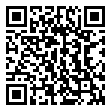• 分享
• 设计
免费设计
• 计算器
装修计算器
• 入驻
合作入驻
• 联系
联系我们
• 置顶
返回顶部